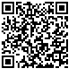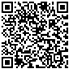## ACT数学必考考点汇总分享，高效复习，才能拿到高分

2019-09-05 10:52:51浏览量：875

ACT数学如何备考?需要掌握哪些方面的知识点?考试过程中会遇到哪些问题?启德考培小编为你介绍ACT数学考试考试内容及问题解析。

ACT数学如何备考?需要掌握哪些方面的知识点?具体的考试内容都有哪些?考试过程中会遇到哪些问题?...如果你被以上问题所困扰的话，一定要继续看完本文。启德考培小编为你介绍ACT数学考试考试内容及问题解析。

ACT数学部分考试时间为60分钟，其中有60道选择题，主要考查的知识点包括六个方面：算术(Pre-Algebra)，初级代数(Elementary Algebra)，中级代数(Intermediate Algebra)，坐标几何(Coordinate Geometry)，平面几何(Plane Geometry)， 三角学(Trigonometry)。

1. 算术(Pre-Algebra)：

考核的内容为高中之前学习的知识。例如，分数(fraction)、小数(decimal)、整数(integer)、平方根(square root)、比率(ratio)、百分比(percent)、整数的倍数(multiple)和因数(factor)、一次方程式(linear equations with one variable)、概率(probability)等。

2. 初级代数(Elementary Algebra)：

考察变量表达式(use variables to express relationships)、代数表达式的代入法(substitute the value of a variable in an expression)、二次方程式的因式分解(solve simple quadratic equations by factoring)、解含有一个变量的线性不等式(solve linear inequalities with one variable)、应用指数和平方根(apply properties of integer exponents and square roots)等。

3. 中级代数(Intermediate Algebra)：

4. 几何坐标(Coordinate Geometry)：

考察标准x，y坐标平面(standard (x ，y) coordinate plane)中的点(point)、线(line)、多项式(polynomial)、圆(circle)、曲线(curve)、斜率(slope)、平行(parallel)、垂直(perpendicular)、距离(distance)、中点(midpoint)、变换(transformation)、二次曲线(conic)等。

5. 平面几何(Plane Geometry)：

考生需掌握平面图形的性质和关系，包括角(triangle)、矩形(rectangle)、平行四边形(parallelogram)、梯形(trapezoid)、圆(circle)、平行线(parallel line)、正交线(perpendicular line)、平移(translation)、旋转(rotation)、周长(perimeter)、面积(area)、体积(volume)等。

6. 三角学(Trigonometry)：

考察直角三角形(right triangle)、三角函数(trigonometric function)的值(value)、属性(property)和图形(graph)、三角恒等式(trigonometric identity)等。

另外，在ACT考试中，考生都会遇到哪些常见问题呢?跟天道小编一起来看。

1、一些考生对题型和考点不是很熟悉，知识点掌握的不全面。在没有进行全面知识点梳理之前，考生不熟悉题目考点，在某一类或者几类题型容易频繁的出现错误。

2、ACT数学考查的是学生的逻辑推理和估算能力，在运算上一般不会特别的复杂，很多考生在基本运算时，由于粗心，很难全作对，这也导致许多遗憾的失分，因此，考生如果想要拿到高分，就需要细心与基本功兼顾。

3、ACT数学的题型比较灵活，许多题目通常会设置背景问题、图标等，学生在紧张的时间面前，对于题目中逻辑关系和关键数据的提取往往不够准确，甚至，有些考生，看到难题就会害怕。

因此，考生在日常备考ACT数学时，需要扎实打好专项词汇基础，做到牢记于心;知识点的把握和难点攻克需要通过系统性学习和充分的刷题来不断提升。

以上就是启德为大家带来的ACT数学考试备考攻略，希望同学们能够高效复习、拿到高分!

## 猜你喜欢## 添加小助手微信## 留学费用查询## 分数兑换器## 雅思/托福测试## 星座对应留学国家

12星座分别适合去哪个国家留学呢，赶快来测测吧